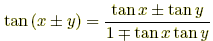index: click on a letter A B C D E F G H I J K L M N O P Q R S T U V W X Y Z A to Z index index: subject areas numbers & symbols sets, logic, proofs geometry algebra trigonometry advanced algebra & pre-calculus calculus advanced topics probability & statistics real world applications multimedia entrieswww.mathwords.com about mathwords website feedback

Distributing Rules

Algebra rules for distributing expressions. See factoring rules as well.

 A. Multiplication 1. addition:  a(b + c) = ab + ac  and  (b + c)a = ba + ca 2. subtraction:  a(b – c) = ab – ac  and  (b – c)a = ba – ca 3. FOIL:  (a + b)(c + d) = ac + ad + bc + bd Careful!! a(bc) ≠ ab·ac B. Division 1. addition: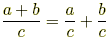2. subtraction: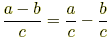Careful!!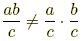C. Exponents   (a ≥ 0, b ≥ 0) 1. multiplication:  (ab)x = axbx 2. division: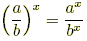(b ≠ 0) Careful!! (a + b)x ≠ ax + bx (a – b)x ≠ ax – bx D. Roots   (x ≥ 0, y ≥ 0) 1. multiplication: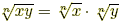2. division: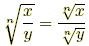( y ≠ 0) Careful!!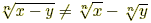E. Logarithms   (x > 0, y > 0, a > 0, a ≠ 1) 1. multiplication:  loga (xy) = loga x + loga y 2. division: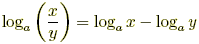3. powers:  loga (xp) = p loga x Careful!! loga (x + y) ≠ loga x + loga y loga (x – y) ≠ loga x – loga y F. Trig 1.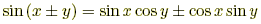2.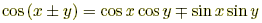3.